# Özetler

A Certain Functional Identity Involving Inverses in Division Rings

Çağrı Demir, Ege Üniversitesi

Abstract: Let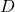be a division ring and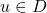a fixed element of. Let further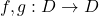be two additive maps satisfying the functional identity

(1)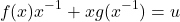for every nonzero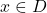. In the talk, we will completely determine all possible forms of the additive maps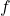and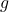that satisfy (1). Our result generalizes some earlier results obtained in  and . If time permits, we will also consider (1) in the context of unital local rings.

This is a joint work with Nefise Cezayirlioğlu.

References:

 Nurcan Argaç, M. Pınar Eroğlu, Tsiu-Kwen Lee, and Jheng-Huei Lin.
Identities with inverses on matrix rings. Linear Multilinear Algebra, 2019,
doi:10.1080/03081087.2019.1575331.
 Louisa Catalano. On a certain functional identity involving inverses. Comm. Algebra,
46(8):3430–3435, 2018.

On Commutator Rings

Münevver Pınar Eroğlu, Dokuz Eylül Üniversitesi

Abstract: For a ring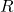and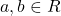, let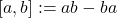, the additive (Lie) commutator of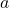and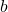. Given two subsets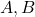of a ring, let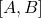denote an additive subgroup ofgenerated by all elements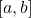for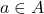and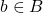. A ringis called a commutator if every element of the ring is a sum of additive commutators; that is,is called a commutator if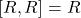, and called a noncommutator if it is not a commutator. For a ringand a positive integer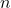, let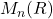be the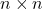matrix ring with coefficients in. If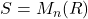is a commutator ring, then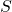is called a commutator matrix ring. In this talk, our goal is to characterize commutator matrix rings by investigating their relationship with commutator rings.

Classification of Ideals and Finite Dimensional Quotients in Leavitt Path Algebras

Suat Sert, Düzce Üniversitesi

Abstract: Leavitt path algebras that were constructed on a field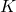and a directed graph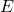, were first defined in 2005, and have since become a popular research topic in both functional analysis and ring theory. In ring theory, the classification of ideals is important, because it contains information about the structure of the ring. Although there is no one single paper on the topic, the ideal structure and types of different ideals of Leavitt path algebras have been studied in many different papers. In this survey, published articles, books and theses on the subject were examined, all known results related to two-sided ideal structure have been compiled. More precisely, prime, maximal, primitive ideal structures, structure theorem for graded ideals, finite dimensional quotients of Leavitt path algebras have been studied.

On the Spectrum of the Normalized Laplacian for Signed Graphs

Hande Tunçel Gölpek, Dokuz Eylül Üniversitesi

Abstract: In this study, the normalized Laplacian matrix for signed graphs and derive interlacingresults for its spectrum are considered. In particular, the effects of several basic graph operations, such as edge removal and addition and vertex contraction, on the Laplacian eigenvalues are investigated. Also vertex replication, whereby a vertex in the graph is duplicated together with its neighboring relations is studied. This operation causes the generation of a Laplacian eigenvalue equal to one. Furthermore, we generalize to the replication of motifs,i.e. certain small subgraphs, and show that the resulting signed graph has an eigenvaluewhenever the motif itself has eigenvalue.

On Some Modules and Ring Extensions

Victor Blasco Jimenez, Zaragoza Üniversitesi

Abstract: In this talk we will discuss about the monoid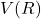of the isomorphism classes of the finitely generated projective modules over a ring. We will use the theory of rings and modules extensions from Bergman’s article in 1974 in order to find rings with good properties for these modules. This will help us to, coming from a finitely generated commutative conical monoid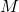with distinguished element different from zero, construct an algebra such that its respective monoidis precisely.

On Stable Equivalence

Zübeyir Türkoğlu, Dokuz Eylül Üniversitesi

Abstract: In this talk, we will give the definition of stable equivalence. Then we will talk about the stable equivalence conjecture and what has been done over Artin Algebras for the following problems.
An old problem: Characterization of stably equivalent Artin algebras.
A weaker form of the old problem: Properties that are invariant under stable equivalence.